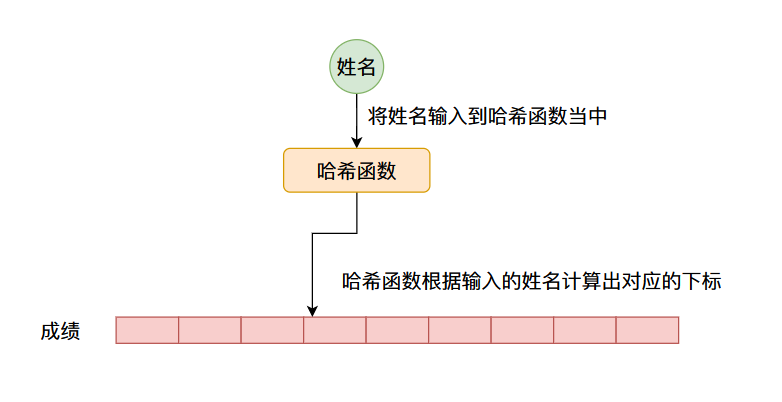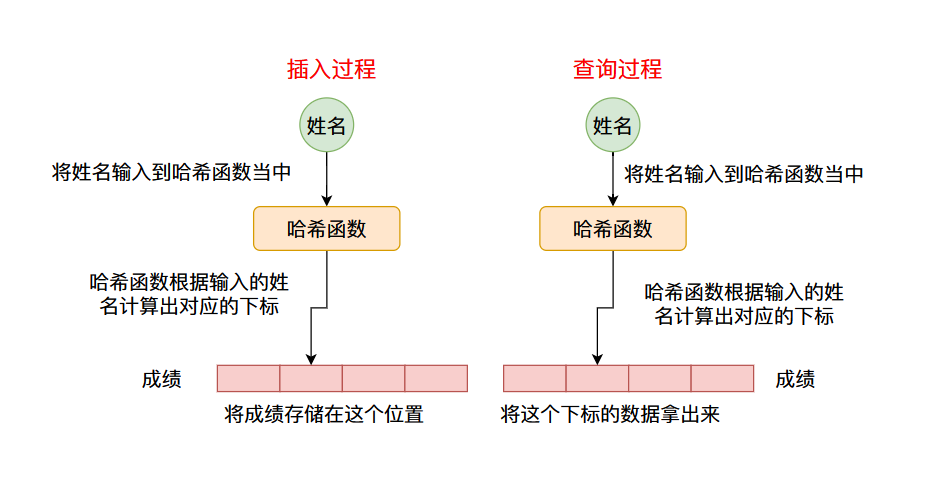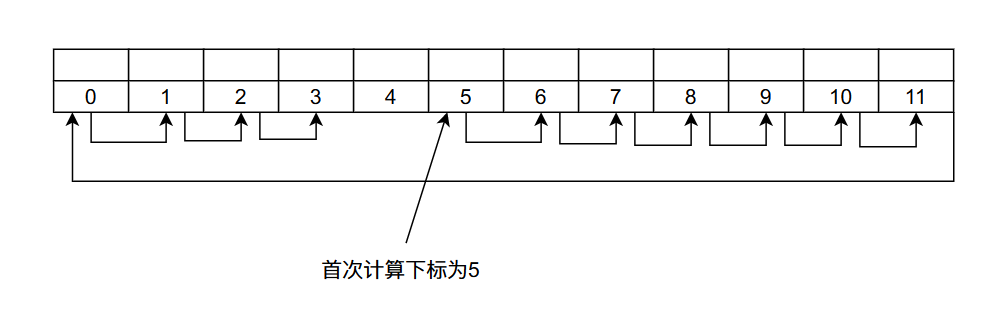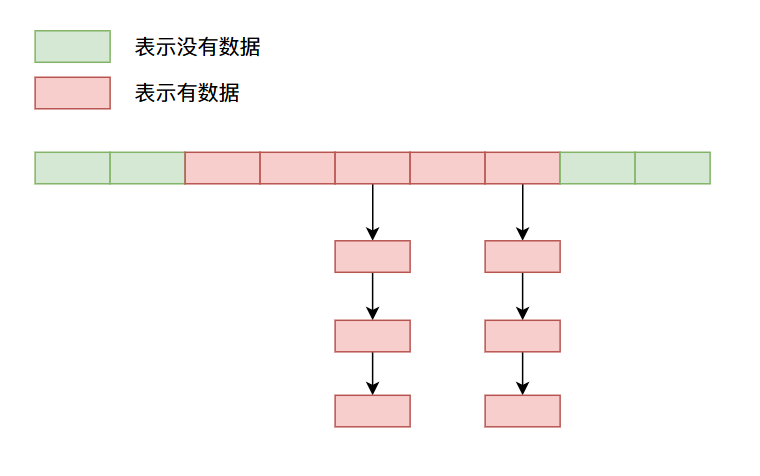# HashMap（Python字典）设计原理与实现（上篇）——哈希表的原理

## HashMap初识

``````  public static void main(String[] args) {
HashMap<String, Integer> map = new HashMap<>();
map.put("学生A", 60);
map.put("学生B", 70);
map.put("学生C", 20);
map.put("学生D", 85);
map.put("学生E", 99);
System.out.println("学生B的分数是：" + map.get("学生B"));
}
``````

``````String[] strs = new String;
strs = "一无是处的研究僧";
System.out.println(strs);
``````

## HashMap整体设计## 如何设计一个哈希函数？

### 整型的哈希函数

``````    /**
* Returns a hash code for a {@code int} value; compatible with
* {@code Integer.hashCode()}.
*
* @param value the value to hash
* @since 1.8
*
* @return a hash code value for a {@code int} value.
*/
public static int hashCode(int value) {
return value;
}

``````

### 字符串的哈希函数

``````public int hashCode() {
// hash 是 String 类当中一个私有的 int 变量，主要作用即存储计算出来的哈希值
// 避免哈希值重复计算 节约时间
int h = hash; // 如果是第一次调用 hashCode 这个函数 hash 的值为0，也就是说 h 值为 0
// value 就是存储字符的字符数组
if (h == 0 && value.length > 0) {
char val[] = value;

for (int i = 0; i < value.length; i++) {
h = 31 * h + val[i];
}
// 更新 hash 的值
hash = h;
}
return h;
}
``````

• 其中`s`，表示存储字符串的字符数组
• `n`表示字符数组当中字符的个数
\[s*31^{(n-1)} + s*31^{(n-2)} + … + s[n-1] \]

### 自定义类型的哈希函数

``````class Student {
String name;
}
``````

``````class Student {
String name;

// 我们自己要实现的哈希函数
@Override
public int hashCode() {
return name.hashCode() * 31 + grade;
}

@Override
public boolean equals(Object o) {
if (this == o) return true;
if (o == null || getClass() != o.getClass()) return false;
Student student = (Student) o;
Objects.equals(name, student.name);
}
}

``````

``````// 下面这个函数是我们自己设计的类 Student 的哈希函数
@Override
public int hashCode() {
}

// 下面这个函数为  Objects.hash 函数
public static int hash(Object... values) {
return Arrays.hashCode(values);
}

// 下面这个函数为  Arrays.hashCode 函数
public static int hashCode(Object a[]) {
if (a == null)
return 0;

int result = 1;

for (Object element : a)
result = 31 * result + (element == null ? 0 : element.hashCode());

return result;
}

``````

`JDK`帮助我们实现的哈希函数，本质上就是将类当中所有的字段封装成一个数组，然后像计算字符串的哈希值那样去计算我们自定义类的哈希值。

### 集合类型的哈希函数

``````public int hashCode() {
int hashCode = 1;
// 遍历集合当中的对象，进行哈希值的计算
for (E e : this)
hashCode = 31*hashCode + (e==null ? 0 : e.hashCode());
return hashCode;
}
``````

\[s*31^{(n-1)} + s*31^{(n-2)} + … + s[n-1] \]

## 哈希冲突

### 开放地址法（再散列法）

#### 线性探测再散列

\[h_i = H(key) \% m \]

\[h_i = (H(key) + d_i) \% m, d_i = i \]#### 二次探测再散列

\[h_i = (H(key) + d_i) \% m, d_i = (-1)^{i – 1} i^2 \]

#### 伪随机数再散列

\[h_i = (H(key) + d_i) \% m, d_i = 一个随机数 \]

### 链地址法## 总结

• `HashMap`整体的设计结构。

• 设计一个好的哈希函数。

• 整型数据的哈希函数。
• 字符串的哈希函数。
• 自定义类型的哈希函数。
• 集合的哈希函数。
• 解决哈希冲突的办法。

• 开放地址法
• 线性探测
• 二次探测
• 随机数探测
• 再哈希法
• 链地址地址法
• 扩容方法